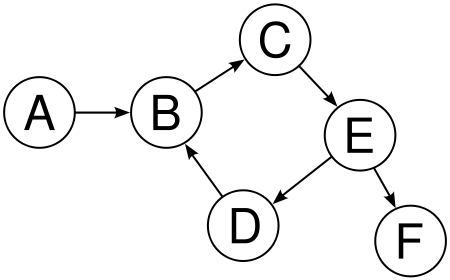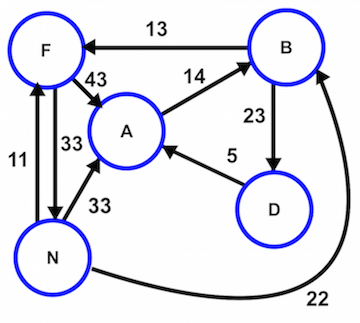## Background

For this homework we are going to explore the world of data structures and algorithms. This is a huge subject and we will only be able to examine a tiny subset of a tiny subset of this area. Specifically, we will focus on the mathematical concept of a graph - a collection of nodes (vertices) some subset of which are connected to one another by edges. For this homework we will specify a simple data structure in R to represent these graphs and you will be responsible for implementing a number of common and useful algorithms for working with this kind of data.

### Graphs

• A graph G = (V,E) is simply a set of vertices V, and a set of edges E between those vertices. It may be more convenient to think about a graph as being a network.

• Edges in graphs can be directed or undirected, the difference being whether the relationship is mutual or one-sided.

• Edges can be weighted, the value of the weight represents some quantitative measure. Most often these weights are thought of as being the distance between the connected vertices.

• A path is a sequence of edges that connects two vertices. There may be many paths, or no paths, between two vertices in a graph.

### Data Structure

We will simplify things somewhat by using only a single data structure for the most general case - a labeled and weighted directed graph. We will represent this as a list of lists in R. The primary list will consist of the graphs vertices which are identified by their indices (position in the list). They may additionally be identified by assigning each element a character string label via the names attribute.

The secondary lists stored in primary list contain the properties of each vertex, specifically an integer vector named edges which stores the indices of vertices connected to the current vertex. Since our data structure is for a directed graph, we will assume that all of these connects are from the current vertex to the listed vertices. It is allowed to have edges that connect a vertex back to itself. The second element for each secondary list is a numeric vector named weights that contains the weights of each connection listed in the edges vector. We again assume that all edges are weighted, if a weight is not specified it should be assumed to be 1 and that all weights should be strictly greater than 0.

Below are example representations of the two graphs at the top of this document:

graph1 = list(A = list(edges   = c(2L),
weights = c(1) ),
B = list(edges   = c(3L),
weights = c(1) ),
C = list(edges   = c(5L),
weights = c(1) ),
D = list(edges   = c(2L),
weights = c(1) ),
E = list(edges   = c(4L,6L),
weights = c(1,1)  ),
F = list(edges   = c(),
weights = c()))
str(graph1)
## List of 6
##  $A:List of 2 ## ..$ edges  : int 2
##   ..$weights: num 1 ##$ B:List of 2
##   ..$edges : int 3 ## ..$ weights: num 1
##  $C:List of 2 ## ..$ edges  : int 5
##   ..$weights: num 1 ##$ D:List of 2
##   ..$edges : int 2 ## ..$ weights: num 1
##  $E:List of 2 ## ..$ edges  : int [1:2] 4 6
##   ..$weights: num [1:2] 1 1 ##$ F:List of 2
##   ..$edges : NULL ## ..$ weights: NULL
graph2 = list(A = list(edges   = c(2L),
weights = c(14)),
B = list(edges   = c(3L,4L),
weights = c(23,13)),
D = list(edges   = c(1L),
weights = c(5) ),
F = list(edges   = c(1L,5L),
weights = c(43,33)),
N = list(edges   = c(1L,2L,4L),
weights = c(33,22,11)))
str(graph2)
## List of 5
##  $A:List of 2 ## ..$ edges  : int 2
##   ..$weights: num 14 ##$ B:List of 2
##   ..$edges : int [1:2] 3 4 ## ..$ weights: num [1:2] 23 13
##  $D:List of 2 ## ..$ edges  : int 1
##   ..$weights: num 5 ##$ F:List of 2
##   ..$edges : int [1:2] 1 5 ## ..$ weights: num [1:2] 43 33
##  $N:List of 2 ## ..$ edges  : int [1:3] 1 2 4
##   ..\$ weights: num [1:3] 33 22 11

### File Format

One of your tasks will be to implement functions that serialize the graph data structure to and from a text file. We will be using a simplified version of the DOT language. Each row of the file corresponds to a single directed edge, this is indicated by the name of the from vertex separated from the name of the to vertex by a ->. Each line must be ended by a semi-colon (;) and weights are optionally indicated by appending a [weight=#] before the semi-colon. Complex names may be escaped using double quotes.

Therefore to indicate an edge between vertices A and B with a weight of 7.2 we would use:

A -> B [weight=7.2];

In order to indicate a vertex with no incoming or outgoing edges a line may contain a single isolated name, e.g.:

M;

Since there is no edge associated with such a line, it is invalid to include [weight=#] on these lines. When creating these files if vertex names do not exist then the index numbers of the vertices should instead be used.

Examples of the files that correspond to the two graphs above are:

# graph1.dot
A -> B;
B -> C;
C -> E;
D -> B;
E -> D;
E -> F;
# graph2.dot
A -> B [weight=14];
B -> F [weight=13];
B -> D [weight=23];
D -> A [weight=5];
F -> A [weight=43];
F -> N [weight=33];
N -> A [weight=33];
N -> B [weight=22];
N -> F [weight=11];

## Functions

Below are a list of functions that you will need to implement for this assignment. For each function you will be given a name, the input arguments, the output type, and a description of the required behavior of the function. Your implementation of these functions may only use R’s base functionality, meaning you are not allowed to directly use any additional packages in your implementation. However, you may use other packages for testing purposes.

Be aware that there are we established algortihms to solve most of the problems listed below - don’t try to reinvent the wheel use what is known to work. Your task is translate the existing algorithms to our specific data structure and R.

### Predicates

• Function - is_valid

• Input - g, a graph object.

• Output - true if valid, false if not.

• Description - Validate the graph object to ensure that it meets all requirements - Check that object is a list of lists. Check if there are names for the primary list that they are all unique. Check that each secondary list contains only edges and weights vectors that are of the appropriate type. Check that there are not any edges to non-existent vertices. Check that all weights are not less than or equal to 0. Check that every edge has a weight.

• Function - is_undirected

• Input - g, a graph object.

• Output - true if undirected, false if not.

• Description - Check if the graph object is undirected, this is true if all directed edges have a complementary directed edge with the same weight in the opposite direction.

• Function - is_isomorphic

• Input - g1, a graph object; g2, a graph object.

• Output - true if g1 and g2 are isomorphic, false if not.

• Description - Check if the graph objects are isomorphic, meaning all vertices, edges, and weights are identical. Comparison of vertices should be based on names not indexes, indexes should only be used if vertex labels are not defined.

• Function - is_connected

• Input - g, a graph object; v1, a vertex label in g; v2, a vertex label in g.

• Output - true if there is a path from v1 to v2 in g, false if not.

• Description - Determine if there is any path between vertex v1 and vertex v2 in graph g. If v1 or v2 are not in g then throw an error.

### Input / Output

• Function - write_graph

• Input - g, a graph object; file, the file to write to.

• Output - None

• Description - Write graph (edges) to the given file according to the file format specification given above.

• Function - read_graph

• Input - file, the file to read from.

• Output - a graph object.

• Description - Read the lines in file and construct the appropriate graph object. If the file violates the specified format throw an error.

### Shortest Path

• Function - shortest_path

• Input - g, graph object; v1, a vertex label in g; v2, a vertex label in g.

• Output - a vector of the names (or indexes if unlabeled) of vertexes that make up the shortest path, in order.

• Description - Find the shortest path from vertex v1 to vertex v2 using the edges of graph g. Note that there may not be a unique solution for any given graph, you are only required to return one path.

### Minimum Spanning Tree

• Function - min_span_tree

• Input - g, graph object.

• Output - a graph object (undirected) containing the minimum spanning tree

• Description - A tree is an undirected graph in which any two vertices are connected by exactly one path (no simple cycles). Therefore, a minimum spanning tree is the tree that connects all vertices in a graph with the shortest possible total of edges, using the existing edges. If given a directed graph return an error. Note that there may not be a unique solution for any given graph, you are only required to return one tree.

### Plotting (Extra Credit)

Come up with an algorithm to plot a graph showing all vertexes (labeled), edges, and weights. Distances between vertexes should be based on edge weights.

## Testing

As this and next week progresses I will be adding tests (using the testthat package) for these functions to every team’s repository. We will additionally be using the Travis continuous integration tool, so that you will be given real time feedback on your progress via github. This process and these tools will be discussed in more detail in class on October 29th.

## Submission and Grading

This homework is due by midnight Sunday, November 9th. You are to complete the assignment as a group and to keep everything (code, write ups, etc.) on your team’s github repository (commit early and often). All team members are expected to contribute equally to the completion of this assignment and group assessments will be given at its completion - anyone judged to not have sufficient contributed to the final product will have their grade penalized. While different teams members may have different coding backgrounds and abilities, it is the responsibility of every team member to understand how and why all code in the assignment works.